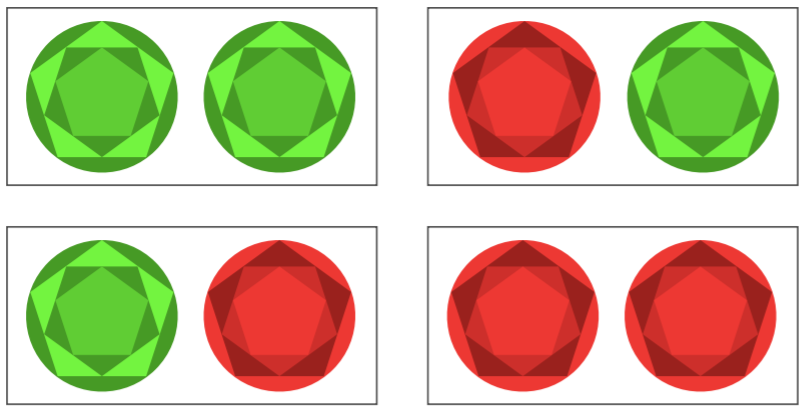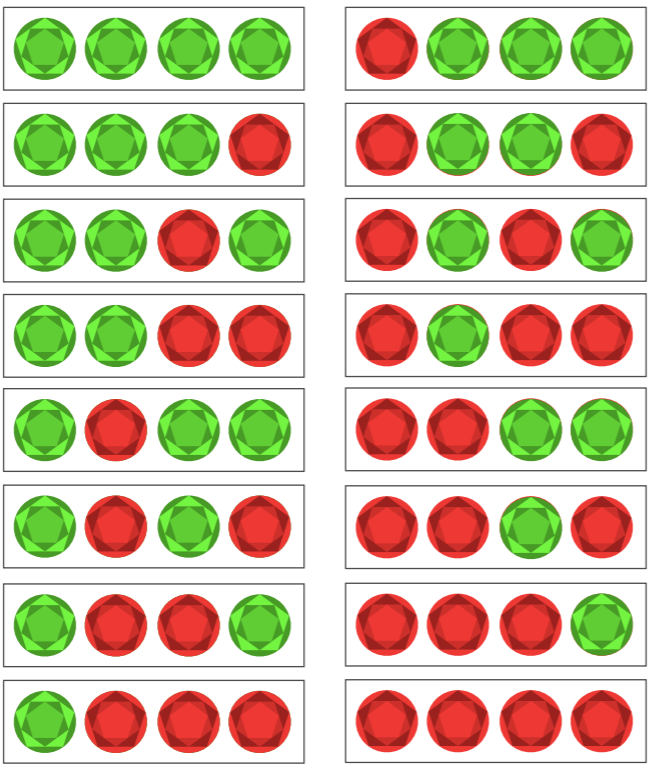#### You may also like### Stop or Dare

All you need for this game is a pack of cards. While you play the game, think about strategies that will increase your chances of winning.### Snail Trails

This is a game for two players. You will need some small-square grid paper, a die and two felt-tip pens or highlighters. Players take turns to roll the die, then move that number of squares in a straight line. Move only vertically (up/down) or horizontally (across), never diagonally. You can cross over the other player's trails. You can trace over the top of the other player's trails. You can cross over a single trail of your own, but can never cross a pair of your trails (side-by-side) or trace over your own trail. To win, you must roll the exact number needed to finish in the target square. You can never pass through the target square. The game ends when a player ends his/her trail in the target square, OR when a player cannot move without breaking any of the rules.### Game of PIG - Sixes

Can you beat Piggy in this simple dice game? Can you figure out Piggy's strategy, and is there a better one?

# Flippin' Discs

##### Age 11 to 14Challenge Level

Ruby and Emma from Yewdale Primary in the UK estimated the numbers of wins they would get by thinking about winning combinations:
2 discs- out of 1000 we got 490 wins which is 49%- We predicted 45% to 55% as there are 4 possibilities and only 2 of them give you a point.

Mahdi from Mahatma Ghandi International School in India showed the 4 combinations as an image, and calculated a probability:From the 4 possible outcomes only 2 are valid.

$\text{Probability} = \dfrac{\text{favourable outcomes}}{\text{total number of outcomes}} = \dfrac24 = 0.5 = 50\%$

Atharva from Wilson's School used the same method, and concluded:
So you should win 50% of the time.

This is the rest of Ruby and Emma's answer. They made mistakes finding the numbers of possibilities, but their answer is still correct in principle:
3 discs- out of 1000 we got 311 wins which is 31.1%- We predicted 20% to 30% as there are nine possibilities and again only 2 of them give you a point.

4 discs- out of 1000 we got 134 wins which is 13.4% - we predicted 10% to 20% as there are 16 possibilities and only 2 of them give you a point.

5 discs - out of 1000 we got 54 wins which is 5.4% - we predicted about 4% to 9% as there are 25  possibilities and only 2 give you a point.

The pattern is that whenever the numbers of discs are bigger, the percentage decreases.

We estimated with 6 discs and out of 1000- we predicted about 3% to 4.5% as
there are 36 possibilities and only 2 give you a point.

Sanika P from PSBBMS, OMR in India, Mahdi and Atharva all continued listing the possibilies. This is Sanika's work for 3 discs:
D1 D2 D3
R R R
G G R
R G R
G R R
R R G
G G G
R G G
G R G

Among these, the first and the sixth (2) combinations are considered as wins out of the 8 possible outcomes.

Therefore the probability of winning when there are 3 discs is $\frac28$ or 25%

This is Mahdi's work for 4 discs:From the 16 possible outcomes only 2 are valid.

$\text{Probability} = \dfrac{\text{favourable outcomes}}{\text{total number of outcomes}} = \dfrac2{16} = 0.125 = 12.5\%$

Atharva spotted a pattern:
2 discs = 50%
3 discs = 25%
4 discs = 12.5%
5 discs = 6.25%

Ergo you just half the percentage for the next one, e.g.
6 discs = 3.125%

Can you see how this follows from Mahdi's image? If you ignore the last disc in each image, the possibilities for 3 discs all appear twice - so the number of possibilities doubles each time you add another disc. How is that related to Atharva's pattern?

Ahan from Tanglin Trust School in Singapore, Mahdi and Sanika found the same pattern but described it in a different way. Sanika wrote:

By extending this is $n$ discs
$D_1,D_2,...D_n$     Each of the discs can have $2$ possible states - Red or Green. So the total [number of] possible outcomes [is] $2^n$

Among the possible outcomes only $2$ could be win wherein all the discs are of same colour.
Therefore the probability of winning with $n$ discs  is equal to $\dfrac2 {2^n} = \dfrac{1}{2^{n-1}}$